# Three Dimensional Geometry NCERT Solutions : Class 12 Maths

## NCERT Solutions for Class 12 Maths : Three Dimensional GeometryFilter
Filters :
Classes
•
•
•
•
•
•
•
Chapters
•
•
•
•
•
•
•
•
•
•
• 3 More
Exercises
•
•
•
•
•

Doubtnut one of the best online education platform provides free NCERT Solutions of Maths for Class 12 Three Dimensional Geometry which are solved by our maths experts as per the NCERT (CBSE) guidelines. We provide the solutions as video solutions in which concepts are also explained along with answer which will help students while learning , preparing for exams and in doing homework. These Solutions will help to revise complete syllabus and score more marks in examinations.

Get here free, accurate and comprehensive NCERT Solutions for Class 12 Maths Three Dimensional Geometry which have been reviewed by our maths counsellors as per the latest edition following up the CBSE guidelines. We provide video solutions in which solutions to all the questions of NCERT Class 12 Maths textbook are explained in step by step and detailed way.

## The Topics of the Chapter Three Dimensional Geometry are :

DIRECTION COSINES AND DIRECTION RATIOS

TYPES OF VECTORS

EQUATION OF LINE PASSING THROUGH TWO GIVEN POINT

VECTOR JONING TWO POINTS

ANGLE BETWEEN TWO LINES

PROPERTIES OF DOT PRODUCT

SHORTEST DISTANCE BETWEEN TWO STARIGHT LINES

DISTANCE BETWEEN SKEW LINES

ANGLE BETWEEN LINE AND PLANE

INTERSECTION POINT OF LINE AND PLANE

DISTANCE BETWEEN PARALLEL PLANES

PLANE

INTRODUCTION

ANGLE BETWEEN TWO PLANES

DISTANCE OF A POINT FROM A PLANE

LINE AND PLANE

EQUATION OF A PLANE BISECTING THE ANGLE BETWEEN TWO PLANES

DISTANCE BETWEEN PLANE

FAMILY OF PLANES

It contains these exercises along with solved examples. All the exercises are solved in the video. Select the exercise to view the solutions exercisewise of the Chapter Three Dimensional Geometry:

## NCERT Solutions Class 12 Maths Chapter Three Dimensional Geometry Exercises:

We have covered all the exercises and also Solved examples in the videos. Along with the practise exercise Students should also practise solved examples to clear the concepts of the Three Dimensional Geometry. If incase you have any doubt you can watch the solutions for the given questions.Watch the solutions for the given questions in which it is also explained in the video steps to solve the questions along with answers.

# NCERT Class 12 Solutions Chapter 11 Three Dimensional Geometry

The topic of three-dimensional geometry is quite important and a bit complicated as compared to its counterpart two-dimensional geometry. One of the ways of describing a 3D object is by assuming its shape as a mesh of triangles. A triangle is generally defined by three vertices wherein the positions of the vertices are described by the coordinates x, y, and z. The major heads that are included in 3D coordinate geometry are the direction ratios and direction cosines of a line segment. Three-dimensional geometry is widely used in various daily life applications such as surveying, astronomy, navigation, crystallography, robotics, building and much more.

Class 12 NCERT Maths book has always been the first preference of students appearing for CBSE class 12 boards. It does not only assist in acquiring knowledge in the mathematical world, but also helpful for the competitive exams. The book is published in such an organized way that it not only makes you stick to the syllabus but also makes your task simpler. The chapter of three-dimensional geometry is explained thoroughly in the NCERT textbook with an easy to understand language followed by simple graphs and diagrams, which makes it quite simpler for students to understand the concepts. NCERT books proves to be quite useful for quick revision while preparing for competitive entrance exams like IIT JEE MAINS and ADVANCED, BITSAT etc.

To add more to its usefulness, students can access Doubtnut at any point in time. At our website, we have provided visuals, graphics, and videos available that are free to access. The use of video tutorials and diagrams with suitable explanations provides ease of learning to the students. In addition, we have one more feature included in our website wherein students and learners can post the photos of the questions from the book and can receive the solution for it. There are several free offerings of doubtnut, some of them are NCERT syllabus, NCERT solutions, NCERT Books, question papers, model question papers, notes, etc. for the convenience of the students.

## Topics & Subtopics of NCERT Class 12 Maths Chapter 11 – Three-Dimensional Geometry

### THREE-DIMENSIONAL GEOMETRY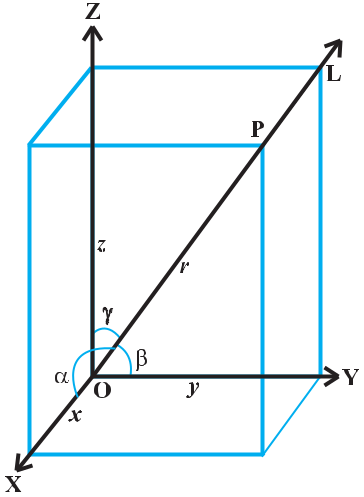A directed line L passing through the origin makes angles α, β, and γ with x, y, and z-axes, respectively, called direction angles, then cosine of these angles, namely, cos α, cos β, and cos γ are called direction cosines of the directed line L. When we reverse the direction of L, then the direction angles are replaced by their supplements, i.e., π α, π β and π γ. So, the signs of the direction cosines are reversed.

Remember: If the given line in space does not pass through the origin, then to find its direction cosines, we draw a line through the origin and parallel to the given line. Now take one of the directed lines from the origin and find its direction cosines as two parallel lines have the same set of direction cosines.

Any three numbers, which are proportional to the direction cosines of a line, are called the direction ratios of the line.

### Direction ratios as direction numbers

Let a, b, c be direction ratios of a line and let l, m and n be the direction cosines (d.c’s) of the line. Then,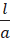=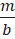=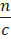= k, k is constant.

 Therefore l = ak, m =bk,n=ck ... (1) But l2 + m2 + n2 = 1 Therefore k2 (a2 + b2 + c2) = 1 Or K=±1a2+b2+c2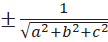Hence, from (1), the d.c.’s of the line is    l = ±aa2+b2+c2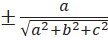,  m = ±ba2+b2+_c2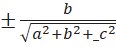n = ± ca2+b2+c2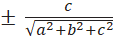For any line, if a, b, c are direction ratios of a line, then ka, kb, kc; k 0 is also a set of direction ratios. So, any two sets of direction ratios of a line are also proportional. Also, for any line, there are infinitely many sets of direction ratios.

### Relation between the direction cosines of a line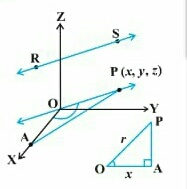Consider a line RS with direction cosines l, m, n. Through the origin draw a line parallel to the given line and take a point P(x,y,z) on this line. From P draw a perpendicular PA on the x-axis.

Let OP = r. Then cos α =OAOP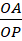= Xl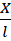So, x = lr.

And,     y = mr, z = nr

Thus,  x2+y2+z2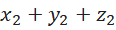= r2(l2+m2+n2)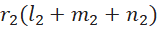But      x2+y2+z2= r2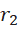Hence    l2+m2+n2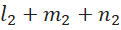=  1

### Direction cosines of a line passing through two points

As only one line passes through two given points, we can determine the direction cosines of a line passing

through the given points P(x1, y1, z1) and Q(x2, y2, z2)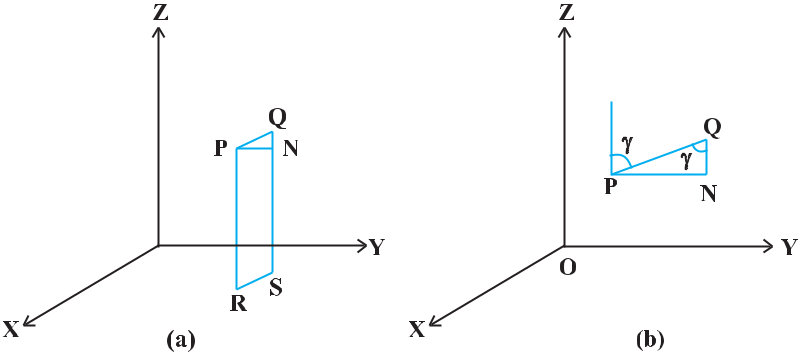Let l, m, n be the direction cosines of the line PQ and let it makes angles α, β, and γ with the x, y, and z-axis, respectively. Draw perpendiculars from P and Q to XY-plane to meet at R and S. Draw a perpendicular from P to QS to meet at N. Now, in right angle triangle PNQ, PQN=γ. So,

Cos γ  =  NQPQ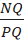=  z2-z1PQ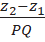, Cos α  =  NQPQ=  x2-x1PQ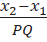, Cos β  =  NQPQ= y2-y1PQ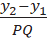PQ  = (x2-x1)2+(y2-y1)2+(z2-z1)2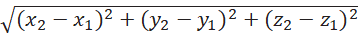### Equation of a Line in Space

We have studied the equation of lines in two dimensions in Class XI, we shall now study the vector and cartesian equations of a line in space. A line is uniquely determined if

• It passes through a given point and has given direction, or
• It passes through two given points.

### Equation of a line through a given point and parallel to a given vector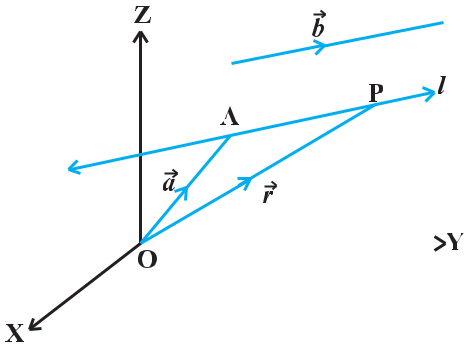Let H be the position vector of the given point A with respect to the origin O of the rectangular coordinate system. Let 1 be the line which passes through point A and is parallel to the given vector b. Let r be the position vector of an arbitrary point P on the line. Then AP is parallel to the vector b i.e.

AP =